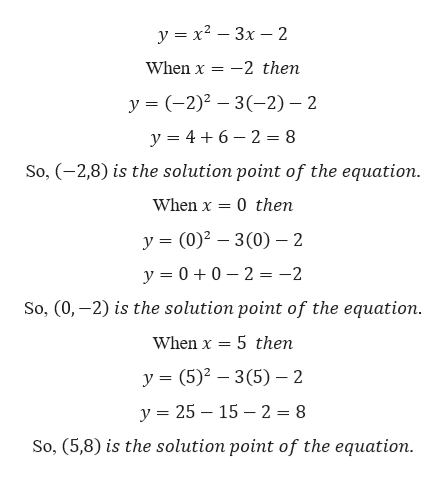# Consider the following equation. y=x2 -3x-2Use the x-values {-2,0 ,5 } to find solution points that should be plotted when graphing this equation. (Select all that apply.)(0,3)(-4,8)(0,-2)(-2,15)(5,8)(-2,8)

Question
7 views

Consider the following equation.
y=x2 -3x-2

Use the x-values {-2,0 ,5 } to find solution points that should be plotted when graphing this equation. (Select all that apply.)

(0,3)

(-4,8)

(0,-2)

(-2,15)

(5,8)

(-2,8)

check_circle

Step 1

Given,

...help_outlineImage Transcriptioncloseу %3D х2 — Зх — 2 When x 2 then у %3 (-2)? — 3(-2) — 2 2 = 8 y 46 So, (2,8) is the solution point of the equation. When x 0 then У %3 (0)2 — 3(0) — 2 y00 2 = -2 So, (0,-2) is the solution point of the equation. When x 5 then у %3 (5)2 — 3(5) — 2 у %3D 25 — 15 — 2%3D 8 So, (5,8) is the solution point of the equation. fullscreen

### Want to see the full answer?

See Solution

#### Want to see this answer and more?

Solutions are written by subject experts who are available 24/7. Questions are typically answered within 1 hour.*

See Solution
*Response times may vary by subject and question.
Tagged in

### Equations and In-equations